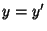## van der Pol Equation

An Ordinary Differential Equation which can be derived from the Rayleigh Differential Equation by differentiating and setting. It is an equation describing self-sustaining oscillations in which energy is fed into small oscillations and removed from large oscillations. This equation arises in the study of circuits containing vacuum tubes and is given by